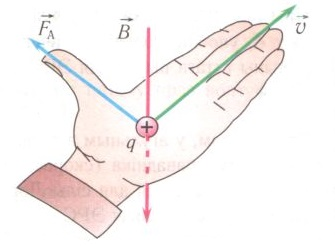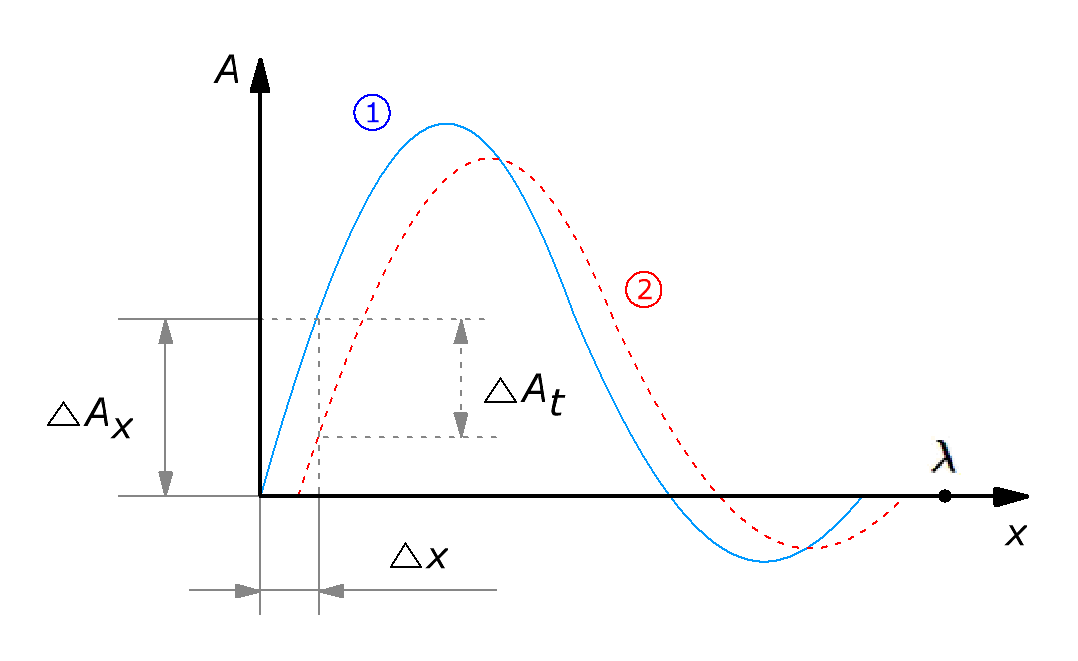Research website of Vyacheslav Gorchilin
2016-09-13
The magnetic component of energy of standing waves
More Amps noticed that when you make the conductor perpendicular to the magnetic field lines in the conductor begins to flow current.Moreover, the velocity vector must be perpendicular as the magnetic field lines and the direction of the current. Ie in fact we have three perpendicularly between the forces passing one another depending on the conditions of the experiment. What if one of the forces, for example magnetic, will form a standing wave, the energy cost of maintaining a minimum? In this work we will try to answer this question.
1. The square of the displacement amplitude of the standing wave
Let's look at two examples example 1 and example 2 In both cases we observe a standing wave, but first wave is visually, and the second moves. In more detail the process of conversion of transverse waves into longitudinal look here.

Since the animation is built with maximum approximation to real lines on the page examples you need to wait for some time until a stationary regime.

For some tasks it is necessary to determine the magnitude of such movement, and as the wave moves across the area, it is obvious that you will find. We agree that further square displacement of a wave we will call PSV, and looking ahead to say that under certain conditions this value — is the numerical expression of so-called free energy. But first we introduce the definition of a wave, and then find her PSV.
The equation of the standing wave
To represent momentum in a long line (DL) will use the standard expression for the falling $$A_1$$ and reflected $$A_2$$ waves . $A_1 = \frac{a}{2} \sin(\omega t - k x), \qquad A_2 = \frac{a}{2} \sin(\omega t + k x) \qquad (1.1)$ in Parallel we will consider a variant of cosine: $A_1 = \frac{a}{2} \cos(\omega t - k x), \qquad A_2 = \frac{a}{2} \cos(\omega t + k x) \qquad (1.2)$ where: $$a$$ is the wave amplitude, $$\omega$$ — the angular frequency, $$t$$ is time, $$k$$ — dimensional multiplier, $$x$$ — distance. As in the further calculations we will use the relative units, the $$\omega = k = 2\pi$$. Also, in these calculations we will assume FOR the ideal, without attenuation. Then the resulting equation of the standing wave will be expressed as $A = A_1 + A_2 = \frac{a}{2} (\sin(\omega t - k x) + \sin(\omega t + k x)) = a\cos(k x)\sin(\omega t) \qquad (1.3)$ $A = A_1 + A_2 = \frac{a}{2} (\cos(\omega t - k x) + \cos(\omega t + k x)) = a\cos(k x)\cos(\omega t) \qquad (1.4)$ the Example described in equation (1.4) waves, which is half-wave DL, is here.
For our purposes we need the complex form of the pulse, therefore, for greater generalization we will present it in the form of the Fourier series. While we consider it a special case — the expansion of the sines and cosines. So, sine and cosine equation of the standing wave in a more General case would be: $A(x,t) = \sum_{i=1}^N (a_i \cos(ik x) \sin(i\omega t)) \qquad (1.5)$ $A(x,t) = \sum_{i=1}^N (a_i \cos(ik x) \cos(i\omega t)) \qquad (1.6)$ where $$N$$ is the number of harmonics $$a_i$$ is the amplitude of the ith harmonic. Now we can represent more complex pulses such as these.
PSV
Next, we need to find the area of the displacement wave which goes on the DL, while moving in time. We denote it by the symbol $$S$$. Its value should be proportional to the displacement of the entire area of the pulse in space and in time. If the momentum in space is fixed, and varies only in time, then the value of $$S$$ must be zero.
The following figure shows the same pulse, but for two different values of time (1) and (2), Pulse forms FOR a standing wave, so the length FOR $$\lambda$$ can take values $$\frac14, \frac12, \frac34, 1$$, etc.We seek the change in the square wave space-time in this form: $\Delta S' = \sum_i {\Delta A_x \over \Delta x } \Delta A_t \qquad (1.7)$ where: $$\Delta A_x$$ — change the amplitude of the pulse at the point $$x$$, $$\Delta x$$ — edit the spatial coordinates in the point $$\Delta A_t$$ is the change in the pulse amplitude when the offset $$\Delta t$$, $$i$$ — the index that goes through all the values of the spatial axis, from zero to $${\lambda \over \Delta x }$$. Then the opposite is true, the expression: $\Delta S' = \sum_i {\Delta A_t \over \Delta t } \Delta A_x$ Turning to the differentials and the integrals, we obtain the following expression: $d S' = \int_0^\lambda {\partial A_x \over \partial x } \partial A_t = \int_0^\lambda {\partial A_x \over \partial x} {\partial A_t \over \partial t} dt \qquad (1.8)$ where: $${\partial A_x \over \partial x} {\partial A_t \over \partial t}$$ — the partial derivatives with respect to spatial and temporal coordinate. Then the total PSV will be true : $S = \iint_0^\lambda {\partial A_x \over \partial x} {\partial A_t \over \partial t} dx\,dt \qquad (1.9)$
Nimirum PSV
To understand what the pulse shape gives a higher PSV, we need to normalize. For this we use a theorem Parseval  for Fourier series and find the quantity surveyor: $\Psi = \iint_0^1 A(x,t)^2 \, dx\,dt \qquad (1.10)$ so now our desired formula will look like this: $\bar S = \frac {S} {\Psi} = \frac {\iint_0^\lambda {\partial A_x \over \partial x} {\partial A_t \over \partial t} dx\,dt} {\iint_0^1 A(x,t)^2 \, dx\,dt} \qquad (1.11)$ Need to say that $$\bar S$$ convenient relative value for comparison. For example, for the classical Tesla transformer , where $$\lambda = \frac14$$ and only one of the first harmonic, $$\bar S = 1$$ (example). So all the other PSV we can compare it with this case. For $$\lambda = \frac12$$ and the first two equal-amplitude harmonics of $$\bar S = 7$$ here it is clearly seen the wave motion in DL (example). If we add harmonics, and this further strengthen the wave motion, it is possible to obtain even greater value $$\bar S$$. So for example this option would give $$\bar S = 64$$.

$$S$$ and $$\bar S$$ will always be taken modulo, as it is important for us the relative and not the absolute value of this quantity. In the formulas, the module is not shown, but implied.

The calculation according to the formula (1.11) easier to carry out the math editor, for example in MathCAD. For this editor and this formula program is here. For $$N=10$$ the editor on a computer with a processor 2.2 GHz, calculates the equation (1.11) for 12 seconds, for $$N=15$$ for 25 seconds. Below we show the simplified formula for a particular case, but which is rendered by the computer in milliseconds.
Half-wave DL
Using the formula (1.11) it is possible to find the relative area of offset for any signal, but since we will only be concerned with pulses in the form (1.5) or (1.6), we can narrow down the problem and to find a more simple equation for a single raspostranenija particular case.
When $$\lambda = \frac12$$ FOR a set of half-wave mode, for which the formula (1.11) obtained a simpler form. For the wave (1.5) the simplified formula would be: $S \approx 8 \sum_{i=1}^{N} a_{i}a_{i-1}{ i^3 (i-1) \over 4i^2-1} \quad \qquad (1.12)$ For the wave (1.6) — like this: $S \approx 8 \sum_{i=1}^{N} a_{i}a_{i-1}{ i^2 (i-1)^2 \over (2i-1)^2} \quad \qquad (1.13)$ They work with an accuracy of 10% in the range $$N \le 12$$. From the formulas, in particular, it follows that $$S$$ is zero if the spectrum of the pulse contains only one harmonic (example, example) or in the spectrum contains only odd harmonics (example). As seen in these examples, wave in FOR not moving. Further summaries will be clear that the odd harmonics are responsible for the steepness of the decline-the rise of the pulse, and even for wave motion in LONG. Also give an example with even and odd harmonics, where it is clearly seen the wave motion.
Nimirum approximate formulas
From formula (1.10) we take the rule of normalization and output it for impulse (1.5) and (1.6): $\Psi = \frac14 \sum_{i=1}^N a_i^2 \qquad (1.14)$ Thus, the total approximate formula for the case of $$\lambda = \frac12$$ and wave (1.5) is this: $\bar S \approx 32 {\sum_{i=1}^{N} a_{i}a_{i-1}{ i^3 (i-1) \over 4i^2-1} \over \sum_{i=1}^N a_i^2} \qquad (1.15)$ and for the wave (1.6) — is this: $\bar S \approx 32 {\sum_{i=1}^{N} a_{i}a_{i-1}{ i^2 (i-1)^2 \over (2i-1)^2} \over \sum_{i=1}^N a_i^2} \qquad (1.16)$ If $$a_{i}$$ are the same, then the formula is quite simplified: $\bar S \approx {32 \over N} \sum_{i=1}^{N} { i^3 (i-1) \over 4i^2-1 } \qquad (1.17)$ $\bar S \approx {32 \over N} \sum_{i=1}^{N} { i^2 (i-1)^2 \over (2i-1)^2} \qquad (1.18)$ From the formulas (1.17, 1.18) implies the interesting fact that the PSV for the sine and cosine waveforms are slightly different.
If the number is equal to the amplitude of the harmonics is large enough $$N \ge 12$$, then the PSV for sine and cosine waveform be approximately equal, and the formula is simplified further : $\bar S \approx \frac43 (2N + 1)(N + 1) \qquad (1.19)$ we must not forget that the approximate formulas (1.12-1.19) derived for the particular case of $$\lambda = \frac12$$, which we will consider in future. For chipremoving pulse PSV calculation is given here.
A game of the mind, or energy pyramid?
This particular case brings us to an interesting observation. In the formula (1.19) was found relative (normalized) value of PSV, but the absolute value of the square displacement will be like this: $S \approx \frac{a^2}{3} N(2N + 1)(N + 1) = 2 a^2 \left({N^3 \over 3} + {N^2 \over 2} + {N \over 6}\right) \qquad (1.20)$ The expression before the parentheses is the square of the wave amplitude or the kind of unit energy, for example, a single block, and in brackets the formula is for building the whole pyramid . In this interpretation $$N$$ is the number of its tiers, and $$S$$ — the total energy of the pyramid.
But our task is to learn to use a PSV to obtain the free energy, which will be discussed in the second part of this article.

The materials used